# 问题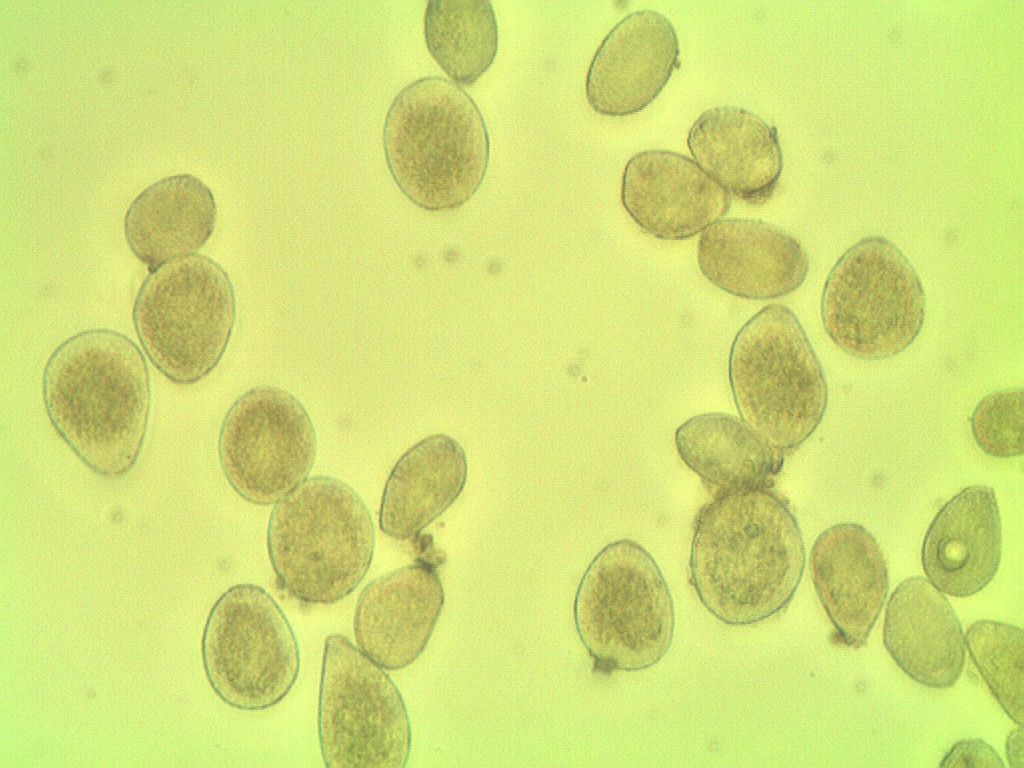# 步骤和Python代码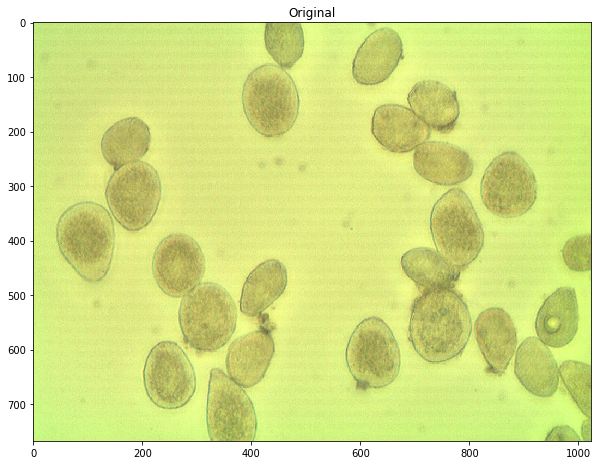Otsu方法是一种自适应阈值化方法，可以用来分割颜色。一般来说，该方法假设可以将图像的像素强度绘制成双峰的直方图，找到灰度直方图的一个最佳的分离点，并以此找到该图的二值化阈值。采取的具体方法为两个部分的类间方差最大，也可以转化为两个部分的类内方差最小。

(而对于假设为单峰的灰度直方图的图像，我们可以采用triangle方法来进行自适应阈值化。)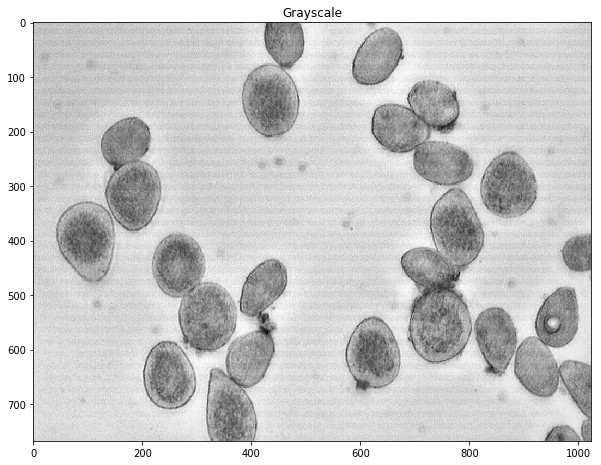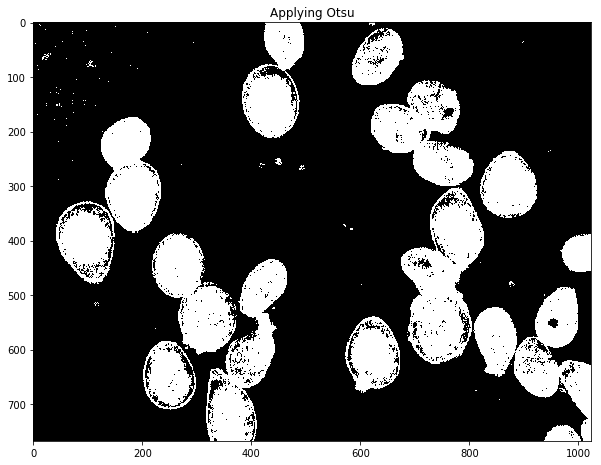（这里也可以通过闭运算、开运算以及其他滤波方法来移除这些噪声，还可以通过计算区域面积来去除小区域，以及利用区域生长的方法来填充孔洞并去除小区域）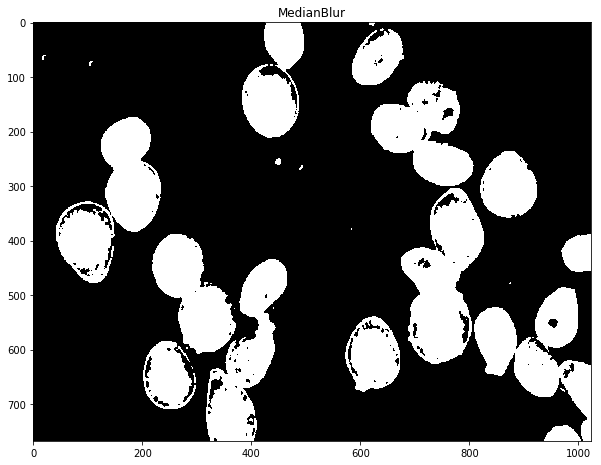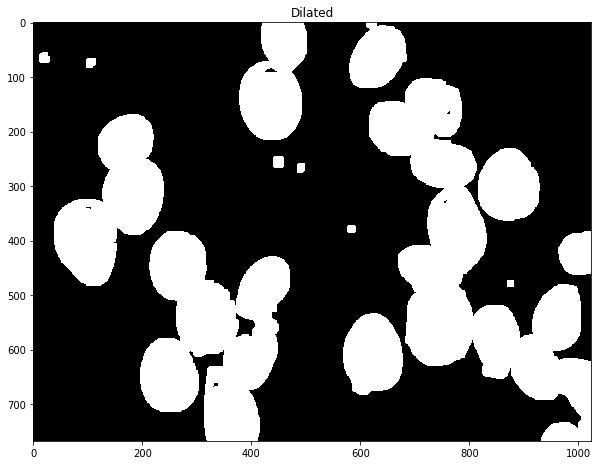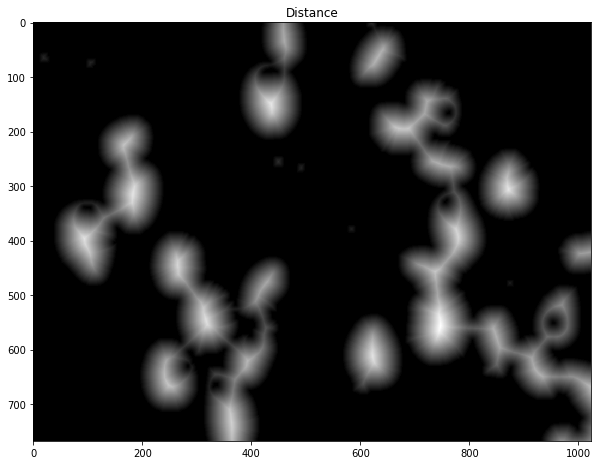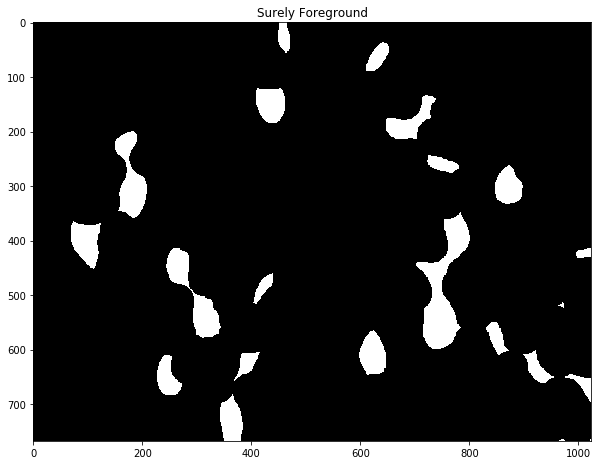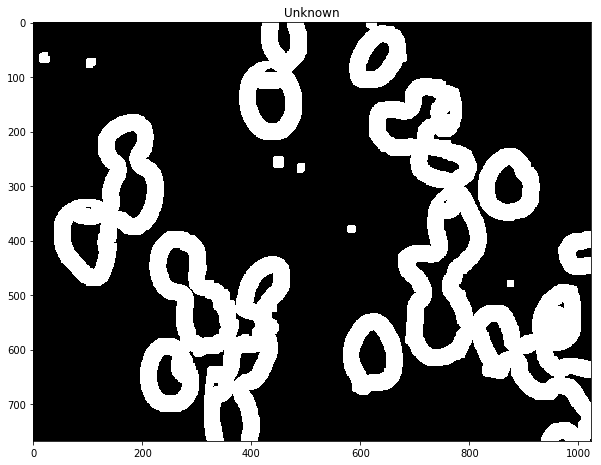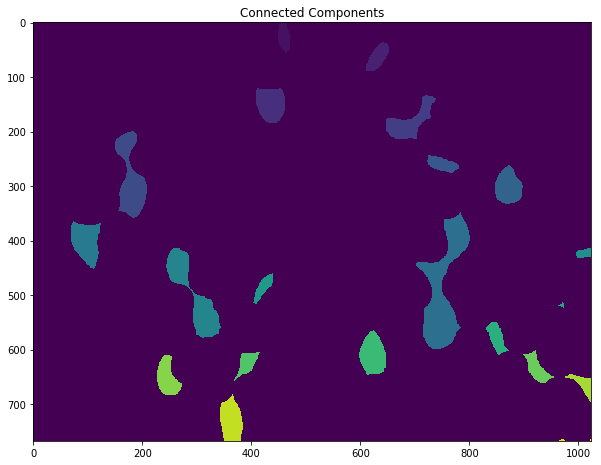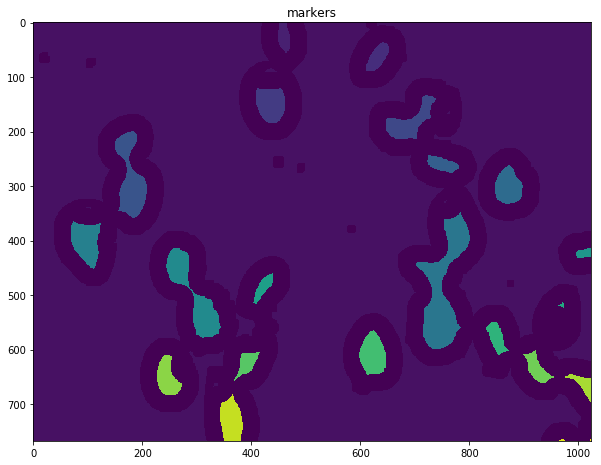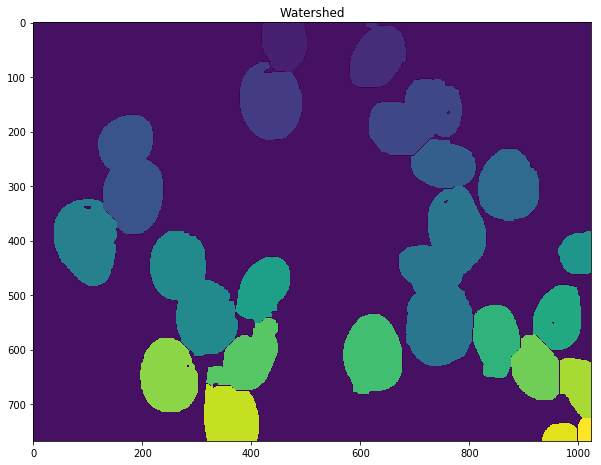23


## 估计不确定性

Dilation=4, FG frac=0.5, Count=22
Dilation=4, FG frac=0.6, Count=23
Dilation=4, FG frac=0.7, Count=17
Dilation=4, FG frac=0.8, Count=12
Dilation=5, FG frac=0.5, Count=21
Dilation=5, FG frac=0.6, Count=23
Dilation=5, FG frac=0.7, Count=20
Dilation=5, FG frac=0.8, Count=13
Dilation=6, FG frac=0.5, Count=20
Dilation=6, FG frac=0.6, Count=23
Dilation=6, FG frac=0.7, Count=24
Dilation=6, FG frac=0.8, Count=14